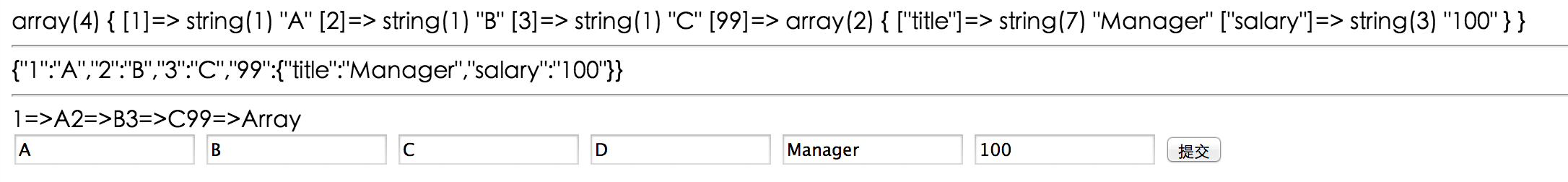# 1. 技术基础

<?php
echo var_dump($_GET['choice']); echo "<hr>"; echo json_encode($_GET['choice']);
echo "<hr>";
$ch =$_GET['choice'];
foreach($ch as$k => $v) { echo$k . "=>" . $v; } ?><form action="test.php" id="form1" name="form1" method="get"> <input type="text" name="choice" value="A" /> <input type="text" name="choice" value="B" /> <input type="text" name="choice" value="C" /> <input type="text" name="choice" value="D" /> <input type="text" name="choice[title]" value="Manager" /> <input type="text" name="choice[salary]" value="100" /> <input type="submit" type="text" name="submit" /> </form> 输出结果：还有两个很重要、很方便的函数：serializeunserialize 多项选择的思路： $ch = $_GET['choice']; foreach($ch as $o) { echo$o;
}

<input type="checkbox" name="choice" value="A" />
<input type="checkbox" name="choice" value="B" checked />
<input type="checkbox" name="choice" value="C" />


# 2. 数据设计

 choice表： choice_id 选项id title 题干 body 选项（数组序列化存储） answer 答案（数组序列化存储） explain 答案解析

 exam表： exam_id 试卷编号 choice_id 题目编号

 exam_session表： user_id 用户id exam_id 试卷编号 mark 试卷得分

 exam_detail表 user_id 用户id exam_id 试卷编号 choice_id 题目编号 user_answer 用户答案

# 4. 参考内容09-17
02-23687
12-165180
09-26296
08-246234
09-02962
07-014279
12-01778